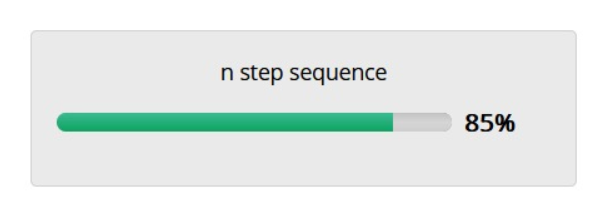## Problem Statement:

Consider a non-empty array inarr consisting of unique integers separated by ‘,'(comma). Write a program to print the output based on the below logic:

Note: inarr will contain at least two elements

###### Input Format :

Read the array inarr having its integers separated by ‘,’ (comma) from the standard input stream

###### Output Format

Print the identified longest n step sequence having its integers separated by “‘(comma) to the standard output stream.

## Question :## Code Answer :

```				```
def solve(s):
l=s.split(",")
for i in range(len(l)):
l[i]=int(l[i])

l.sort()
ans=[l,l]
for i in range(len(l)):
for j in range(i+1,len(l)):
p=[l[i],l[j]]
diff=l[j]-l[i];
u=l[j]
for k in range(j+1,len(l)):
if l[k]-u==diff:
p.append(l[k])
u=l[k]

if len(ans)==len(p):
if ans-ans<p-p:
ans=p
if len(ans)<len(p):
ans=p

w=""
for x in range(len(ans)-1):
w=w+str(ans[x])+','
w=w+str(ans[len(ans)-1])
return w

s=str(input())
print(solve(s))
```
```